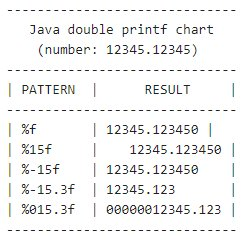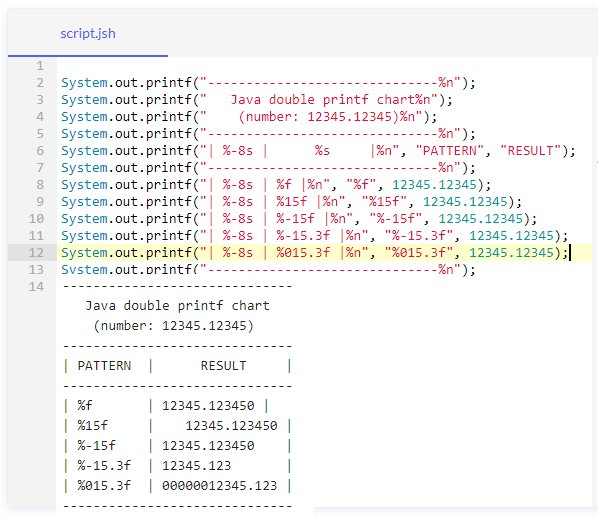# How to format a Java double with printf example

## How use Java printf to format a double’s decimals

To use Java `printf` to format double and float values with decimal place precision, follow these two rules:

1. Use `%f` as the double specifier in the text string.
2. Precede the letter f with a decimal and number to specify precision.

## Java double printf example

Here is a simple example of both a float and double being formatted with Java’s `printf` function:

```package com.mcnz.rps;

public class JavaPrintfDoubleExample {
/* Java double printf code example. */
public static void main(String[] args) {
double mint = 1234.12345;
float  sum  = 1234.12345f;
System.out.printf("%,.3f :: %,.5f", mint, sum);
/* Example prints: 1,234.123 :: 1234.12345 */
}
}```

### Java double printf function explained

There are three key takeaways from this Java `printf` example for decimal places:

• The `%f` specifier works with both double and float values.
• The decimal and number after the % specify the number of decimals to print.
• A comma after the % sign adds a grouping separator for large numbers.

## Is the Java printf specifier %f or %d for floating-point doubles?

Many people confuse the `%d` and `%f` `printf` specifiers.

Both `%d` and `%f` are valid when used with the Java `printf` function. However, only `%f` is intended for use with floating point values such as floats and doubles.This quick chart shows how to use Java printf with double types.

The `%d` specifier is to be used with non-floating point decimal whole-numbers such as byte, short, int and long.

## Java double printf syntax

The format for the Java `printf` function is as follows:

`% [flags] [width] [.precision] specifier-character`
 printf flag Purpose + Will force the number to be preceded by a plus or minus sign 0 Results in zero padding for numbers below the specified width , The grouping separator for large values A blank space will show a minus sign for negative numbers and a space for positive numbers

## Advanced Java decimal printf code example

Here is a more advanced Java `printf` format code example with double values:

```public class AdvancedJavaPrintfDoubleExample {
/* Advanced Java printf double example */
public static void main(String[] args) {
double iLovePie = Math.PI * 999 * -1;
System.out.printf("Value is: %0,10.2f", iLovePie);
/* This printf double example outputs 'Value is: -03,138.45' */
}
}```

The output of this code example which uses %0,10.3f as the format specifier is:

`Value is: -03,138.45`

The %0,10.3f  format specifier breaks down like this:

• The number generated totals 10 characters in width, including the sign, radix and grouping comma.
• Zero padding on the left ensures all 10 characters are used.
• Precision is to two decimal places.
• A comma groups large numbers by the thousands.

## How can Java printf format a double to two decimal places?

It is a common requirement to format currencies to two decimal places.

You can easily achieve this with the Java `printf` function. Just use %.2f as the format specifier. This will make the Java `printf` format a double to two decimal places.

```/* Code example to print a double to two decimal places with Java printf */
System.out.printf("I love %.2f a lot!", Math.PI);```

Without Java `printf`, double formatting is a difficult challenge. But with this simple method built right into the Java API, you can make floating point data easy to read and easy for your users to understand.

## Java double printf table example

The chart above, which shows how different patterns impact the formatting of a double, was actually created with `printf` statements.

Here’s the full code:

```System.out.printf("------------------------------%n");
System.out.printf(" Java double printf chart%n");
System.out.printf(" (number: 12345.12345)%n");
System.out.printf("------------------------------%n");
System.out.printf("| %-8s | %s |%n", "PATTERN", "RESULT");
System.out.printf("------------------------------%n");
System.out.printf("| %-8s | %f |%n",      "%f",      12345.12345);
System.out.printf("| %-8s | %15f |%n",    "%15f",    12345.12345);
System.out.printf("| %-8s | %-15f |%n",   "%-15f",   12345.12345);
System.out.printf("| %-8s | %-15.3f |%n", "%-15.3f", 12345.12345);
System.out.printf("| %-8s | %015.3f |%n", "%015.3f", 12345.12345);
System.out.printf("------------------------------%n");```

Sometimes it’s fun to make charts tables with Java’s `printf` command.Here’s the code and output of a table of Java double printf examples.

Close